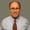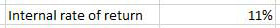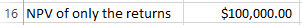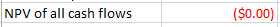# Spreadsheets for Finance: Calculating Internal Rate of ReturnIf you want to choose between several possible investments, a good measuring stick to use is Internal Rate of Return. You could just calculate the plain, vanilla return on investment, commonly known as ROI, but that's simply the investment return divided by cost. Internal rate of return, or IRR, instead looks at cash flows over time from your investment.

That way, if you have an idea of what the cash flows will be for the alternative investments you're evaluating, IRR will give you a side-by-side comparison of your investments. Even if the investments you're comparing don't have the same cash flows every time, you can still calculate their internal rate of return as long as the cash flows are periodic (e.g. every quarter or every year). If the cash flows don't come at regular intervals, you can still use a similar function, called XIRR.

Here's everything you need to know about calculating the internal rate of investment—with periodic or non-regular cash flows—in any version of Excel, Google Sheets, Numbers, or any other standard spreadsheet app.

## Screencast

As with our other Spreadsheets for Finance tutorials, you can follow along in your own spreadsheet app by hand to get extra practice and try out your own numbers while you're following along, or you can download the included spreadsheet file and open it in Excel or any other spreadsheet app to get started quickly.

## Calculating Internal Rate of Return

The internal rate of return (IRR) function requires two parameters and possibly an optional one:

• The initial investment. This should be negative, because it's a cash outflow.
• A list of the periodic returns. These can be positive or negative.
• Optional guess at the result: if the function returns an error, you can help it by guessing what you think the result should be. If you don't guess, the function will guess at 10%.

The syntax for the IRR function is:
=IRR(cash flows, [guess])

Note that the initial investment and the cash flows are all part of the same argument of the function.

IRR is also related to net present value, which is another good evaluation tool. IRR is the discount rate that makes the net present value of the income stream equal to zero. Below, I'll show you how to use that to check the result (be sure to check our tutorial on Calculating Net Present Value as well if you haven't read it already).

Open Internal Rate of Return.xlsx or type in what's in the screen capture below.

We'll compare two alternatives to see which yields a better return. Immediately we see that the second one requires an investment that's 50% higher, the returns are higher, and they all get paid sooner—but not on a regular schedule.

Click in B13 and enter the function:
=IRR(B4:B9)

This shows an internal rate of return of 11%.## When the Returns Don't Come in Regularly

When the returns come in at irregular intervals like in the second example, use the XIRR function.

The XIRR function requires three parameters and possibly an optional one:

• The initial investment: This should be negative, because it's a cash outflow.
• A list of the periodic returns: These can be positive or negative.
• The dates of the returns: These should be formulas, such as the DATE function, or the results of another calculation. Don't enter them manually, because that can yield bad results.
• Optional guess at the result: if the function returns an error, you can help it by guessing what you think the result should be. If you don't guess, the function will guess at 10%.

Now click in E13 and enter the function:
=XIRR(E4:E9,F4:F9)

This shows an internal rate of return of 10%.

## Checking IRR Using the NPV Function

There are two ways of using net present value to check the IRR:

• The NPV of the returns at the rate calculated as the IRR should be equal to the initial investment, expressed as a positive number
• The NPV of all cash flows at the rate calculated as the IRR should be equal to zero

Click in B16 and enter the function:
=NPV(B13,B5:B9)

That should display the initial investment of \$100,000 as a positive number.Click in B17 and enter the function:
=NPV(IRR(B4:B9),B4:B9)

That should display zero.This might display formatted as a negative number. That's simply a quirk of the worksheet rounding off a lot of decimal points. Just ignore it.

## Conclusion

When you want to evaluate the quality of an investment's returns over time, especially if you want to compare the quality of several investments that you might make, an internal rate of return calculation is a good measuring tool. When the returns will come at regular intervals, use the IRR function, and when the returns will come at irregular but predictable intervals, use the XIRR function. These methods will let you make a meaningful comparison of the investments you're considering.